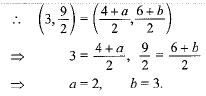Courses

# MCQ : Coordinate Geometry - 1

## 10 Questions MCQ Test Mathematics (Maths) Class 10 | MCQ : Coordinate Geometry - 1

Description
This mock test of MCQ : Coordinate Geometry - 1 for Class 10 helps you for every Class 10 entrance exam. This contains 10 Multiple Choice Questions for Class 10 MCQ : Coordinate Geometry - 1 (mcq) to study with solutions a complete question bank. The solved questions answers in this MCQ : Coordinate Geometry - 1 quiz give you a good mix of easy questions and tough questions. Class 10 students definitely take this MCQ : Coordinate Geometry - 1 exercise for a better result in the exam. You can find other MCQ : Coordinate Geometry - 1 extra questions, long questions & short questions for Class 10 on EduRev as well by searching above.
QUESTION: 1

### If the distance between the points (2, - 2) and (-1, x) is 5, one of the values of x is

Solution:

(5)²= [{2-(-1)}² + {x-(-2)}²]
25= 9+(x+2)²
25-9 = (x+2)²
√16=x+2
x+2= 4
x=4-2
x= 2.

QUESTION: 2

### A triangle with vertices (4, 0), (- 1, - 1) and (3, 5) is a/an

Solution:

Let A(4, 0), B(-1, -1), C(3, 5)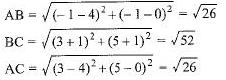⇒ AB2 + AC2 = BC2 and AB = AC
Hence, triangle is an isosceles right-angled triangle.

QUESTION: 3

### The points A (9, 0), B (9, 6), C (-9, 6) and D (-9, 0) are the vertices of a

Solution:

AB= √(9-9)²+(6-0)²=6
BC= √(-9-9)²+(6-6)²=18
CD = √(-9+9)²+(0-6)²=6
DA = √(-9-9)²+(0-0)²=18
so,it's opposite sides are equal,hence it is rectangle

QUESTION: 4

The points (- 4,0), (4, 0) and (0,3) are the vertices of a/an

Solution:

Isosceles triangle.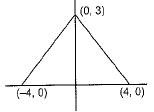QUESTION: 5

The distance between the points A (0, 6) and B (0, -2) is

Solution:

Since both these points lie on a straight line i.e x axis, distance will be the difference between the respective y coordinates
(0,-2) & (0,6)  =>  6-(-2)) = 6+2 = 8

QUESTION: 6

A circle drawn with origin as the centre passes through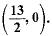The point which does not lie in the interior of the circle is

Solution:

Distance of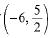from centre of the circle i. e.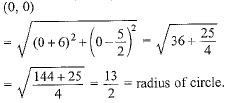QUESTION: 7

The distance between the points A (0, 7) and B (0, -3) is

Solution:

Since both these points lie on a straight line i.e x axis, distance will be the difference between the respective y coordinates

(0,-3)   (0,7)

7-(-3) = 7+3 = 10

QUESTION: 8

If the distance between the points (4,p) and (1,0) is 5 units, then the value of p is

Solution: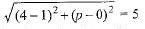⇒ 32 + p2 = 52 ⇒ p2 = 25 - 9 = 16 ⇒ p = ± 4

QUESTION: 9

The end points of diameter of circle are (2, 4) and (-3, -1). The radius of the circle is

Solution:

diameter = √((2-(-3))+ (4-(-1))2)

= √52+52 = √50 = 5√2

QUESTION: 10

If P(1, 2), Q(4,6), R(5,7) and S(a, b) are the vertices of a parallelogram PQRS, then

Solution: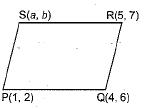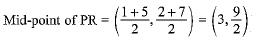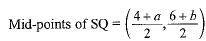Diagonals of parallelogram bisect each other.